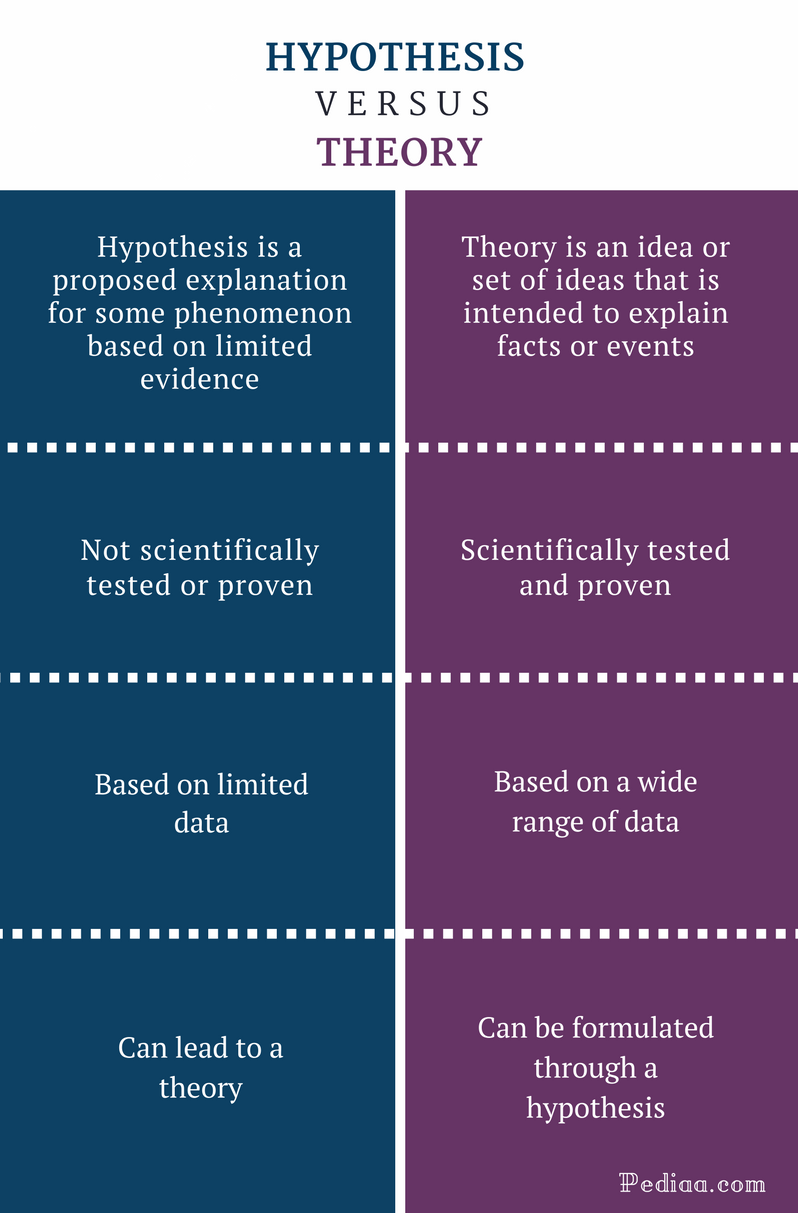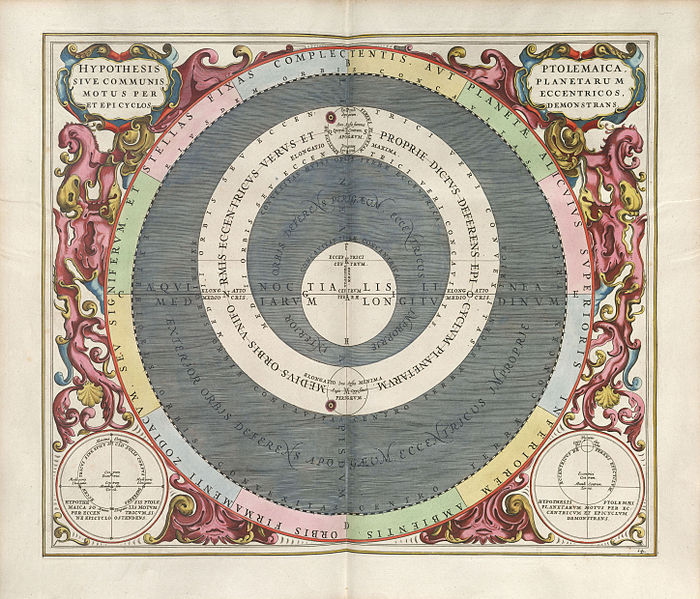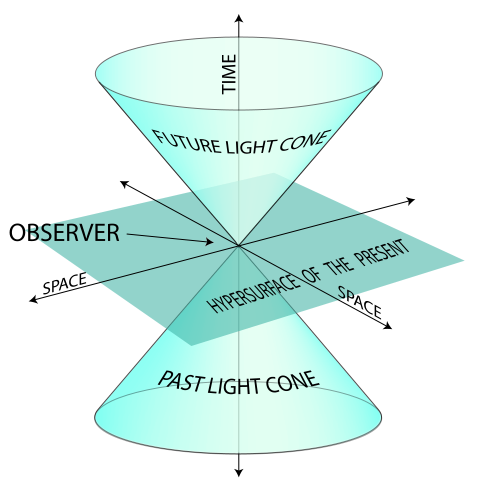# Difference Between Hypothesis and Theory

## Main Difference – Hypothesis vs Theory

Hypothesis and theory are two words that are often used in the field of science and research. Although these two words have somewhat similar meanings, there is a fundamental difference between hypothesis and theory. Hypothesis is a suggested explanation to explain some phenomenon, and is based on limited data. Theory, on the other hand, is a set of ideas that is intended to explain facts or events; they are based on concrete evidence. This is the main difference between hypothesis and theory.

1. What is a Hypothesis? – Definitions and Features

2. What is a Theory? – Definitions and Features

3. What is the difference between Hypothesis and Theory?## What is a Hypothesis

A hypothesis is a proposed explanation based on some evidence.  According to the Oxford dictionary, hypothesis is “a supposition or proposed explanation made on the basis of limited evidence as a starting point for further investigation” and Merriam-Webster dictionary defines it as “an idea or theory that is not proven but that leads to further study or discussion.”

However, a hypothesis is not scientifically tested or proven; it is a logical assumption based on the available evidence. A hypothesis can be accurate or inaccurate. Once the hypothesis is scientifically tested and proven, it becomes a theory.The hypothesis of Andreas Cellarius, showing the planetary motions in eccentric and epicyclical orbits.

## What is a Theory

Theory is an idea or set of ideas that is intended to explain facts or events. A theory is formulated after in-depth research analysis. It is always proven scientifically with evidence. The Oxford dictionary defines theory as “supposition or a system of ideas intended to explain something, especially one based on general principles independent of the thing to be explained.”

As mentioned above, a theory is usually formulated from a hypothesis. Once a hypothesis is tested and proven, it is accepted as a theory. Copernicus’ Heliocentric theory, Darwin’s theory of evolution, quantum theory, special relativity theory, are examples of are some important scientific theories.

A theory can be used to understand, explain and make predictions over a concept. However, theories can be proven to be wrong as well, depending on the proof. However, theoretical knowledge is important in understanding different concepts and situations.Special Theory of Relativity

## Difference Between Hypothesis and Theory

### Definition

Hypothesis is a proposed explanation for some phenomenon based on limited evidence.

Theory is an idea or set of ideas that is intended to explain facts or events.

### Testing and Proof

Hypothesis is not scientifically tested or proven.

Theory is scientifically tested and proven.

### Data

Hypothesis is based on limited data.

Theory is based on a wide range of data.

### Interdependence

Hypothesis can lead to a theory.

Theory can be formulated through a hypothesis.

Image Courtesy:

“Cellarius Harmonia Macrocosmica – Hypothesis Ptolemaica” By Andreas Cellarius – (Public Domain) via Commons Wikimedia

“World line” By SVG version: K. Aainsqatsi at en.wikipediaOriginal PNG version: Stib at en.wikipedia – Transferred from en.wikipedia.(Original text : self-made) (CC BY-SA 3.0) via Commons Wikimedia